# RD Sharma Solutions for Class 8 Maths Chapter 20 Mensuration - I (Area of a Trapezium and a Polygon) Exercise 20.2

This Exercise 20.2 of Chapter 20, deals with the area of a trapezium. Students who find it difficult to solve the problems can refer to RD Sharma Class 8 Solutions which are designed by our expert tutors. Solutions here are prepared in a simple and understandable language which helps students score well in their exams. Students can easily download the pdf from the links provided below and start practising offline.

## Download the Pdf of RD Sharma Solutions for Class 8 Maths Exercise 20.2 Chapter 20 Mensuration – I (Area of a Trapezium and a Polygon)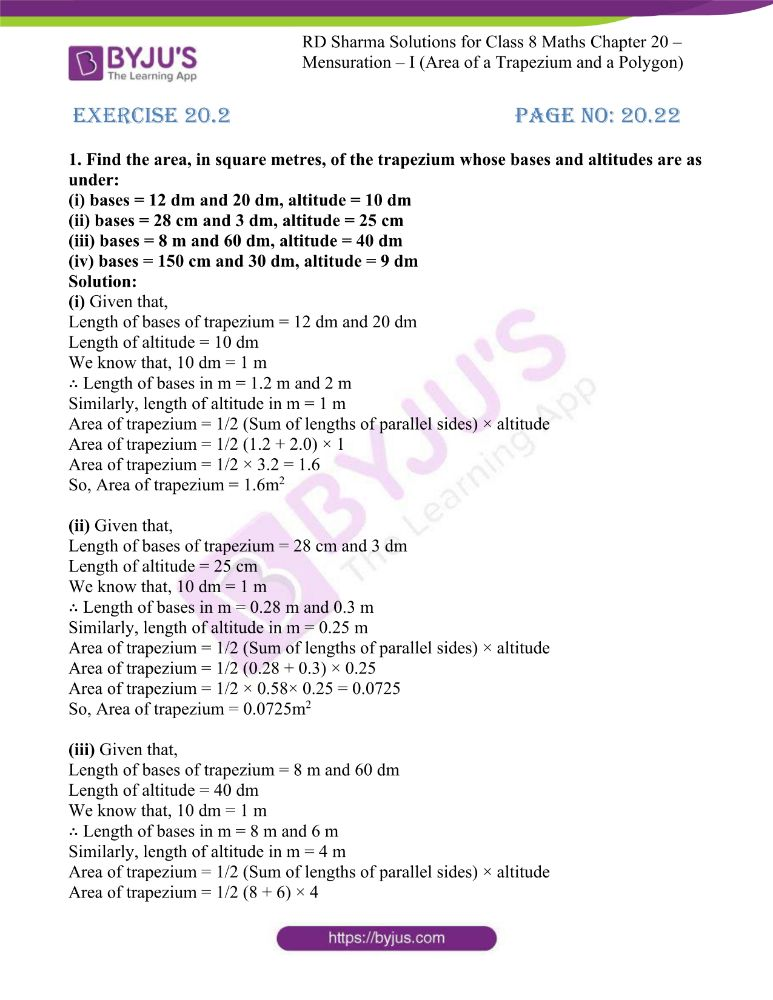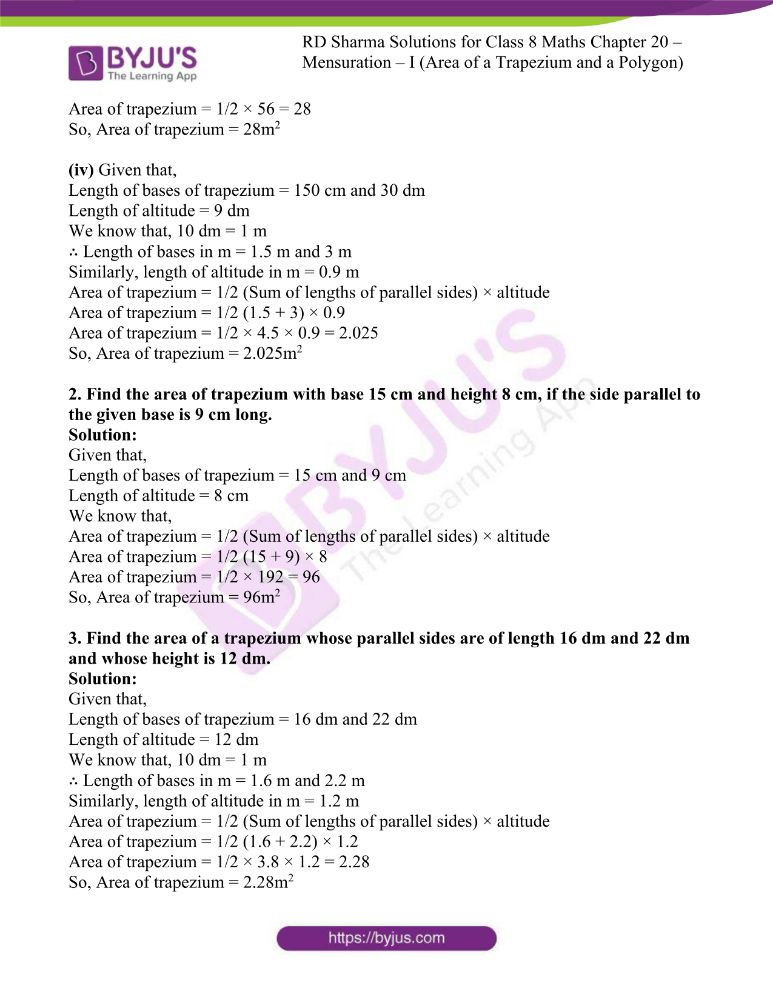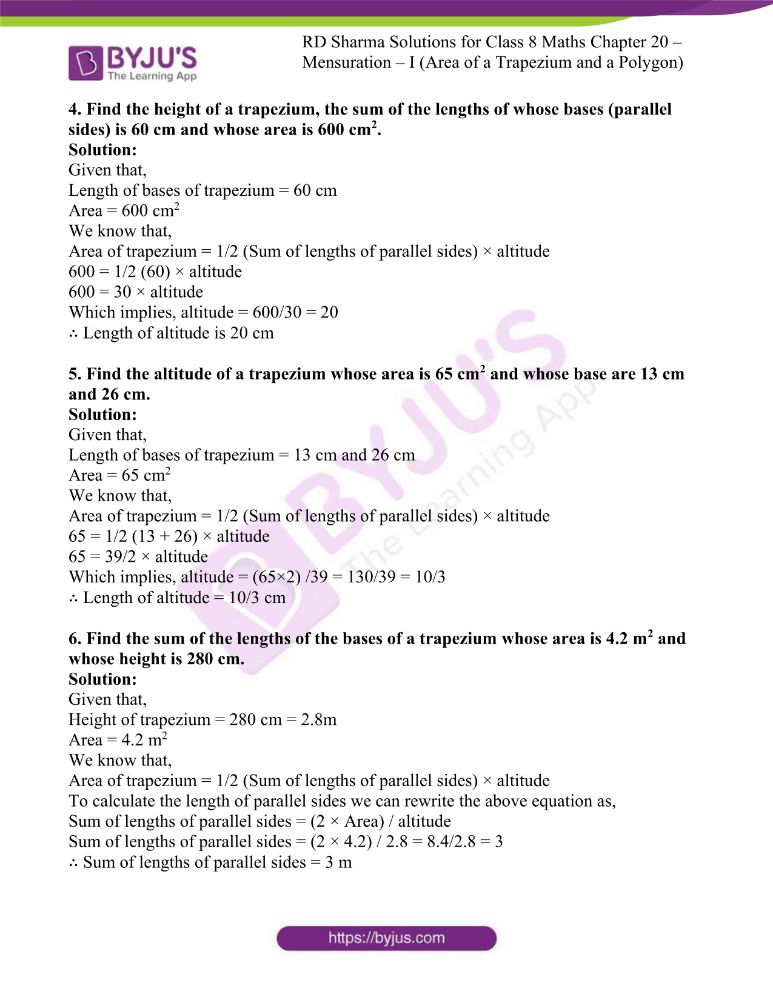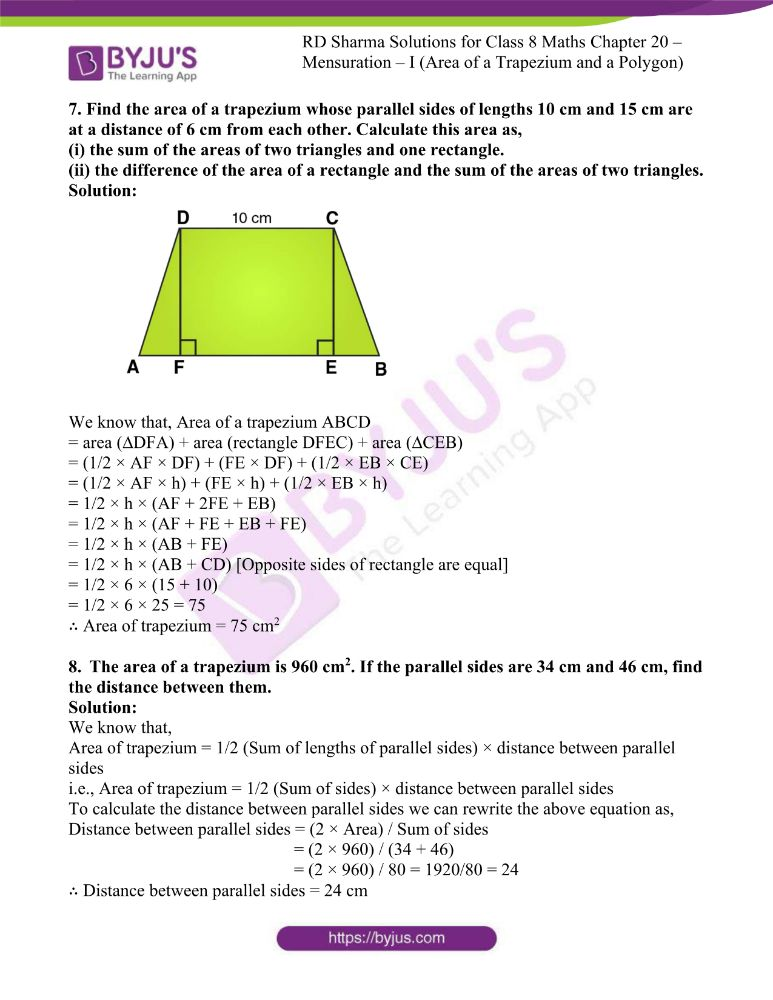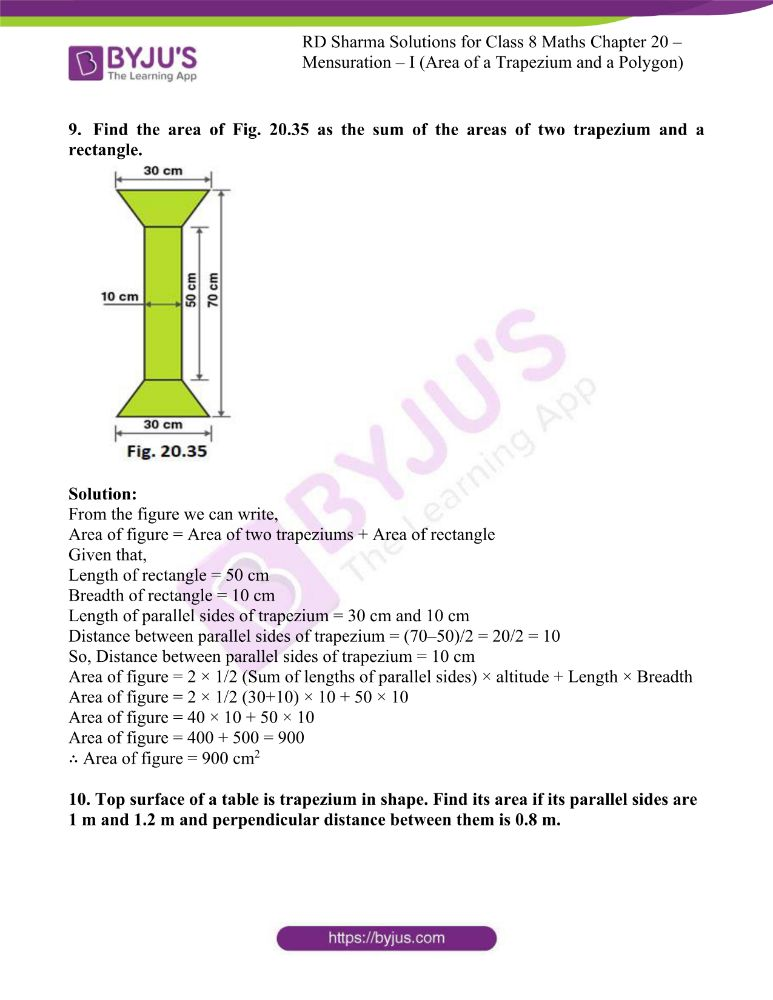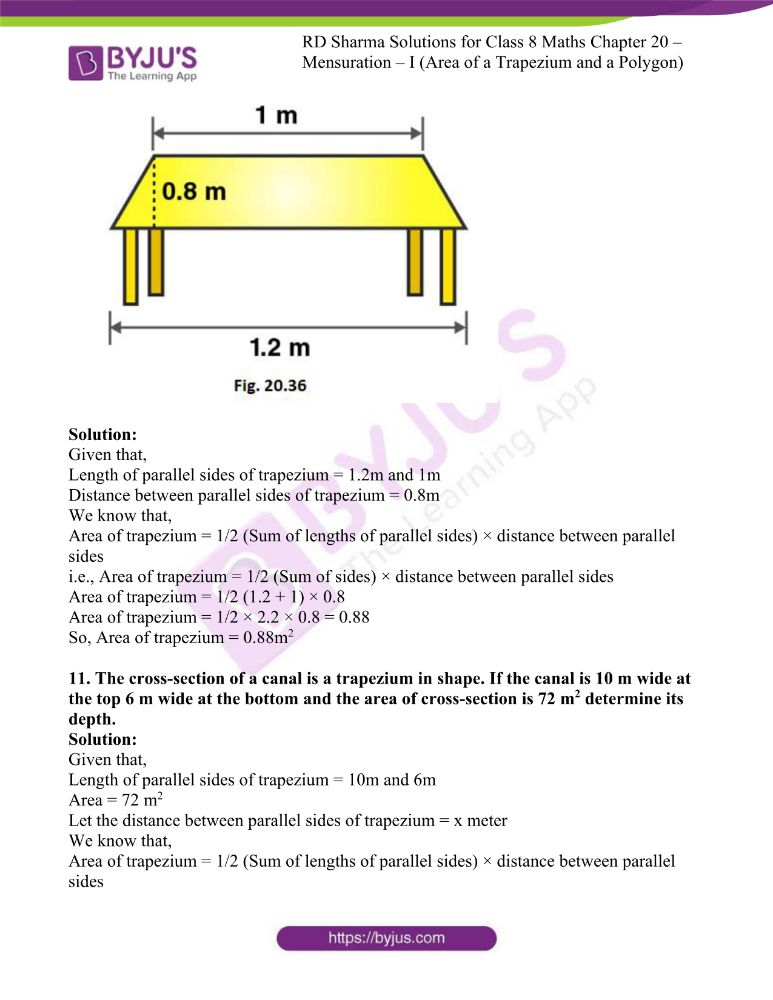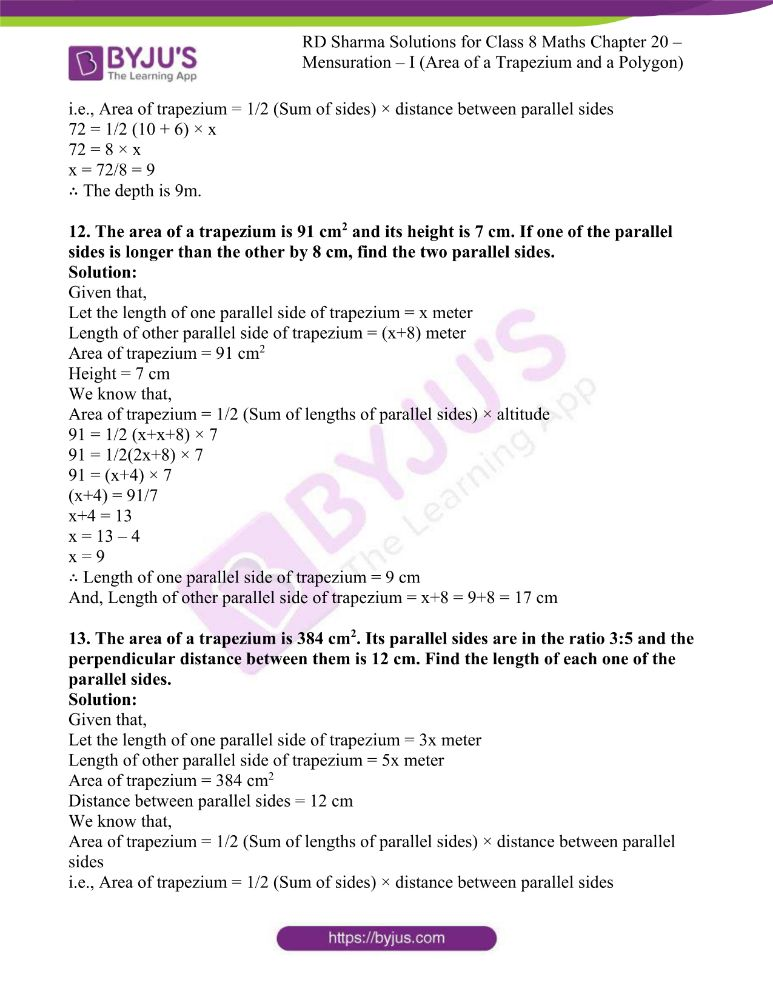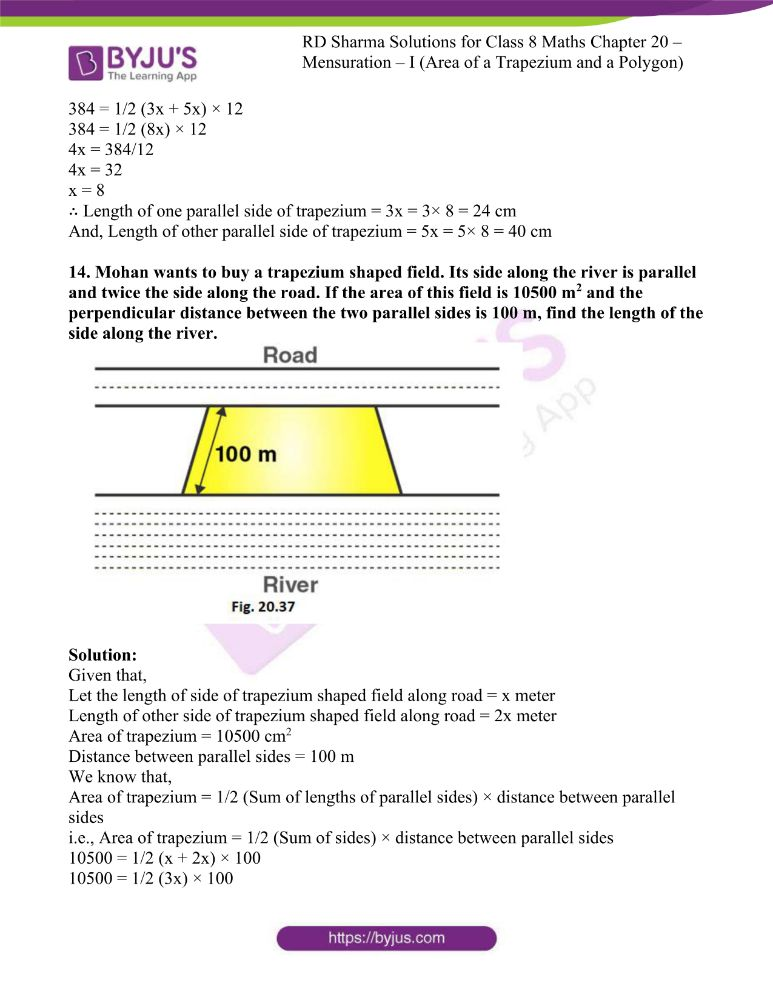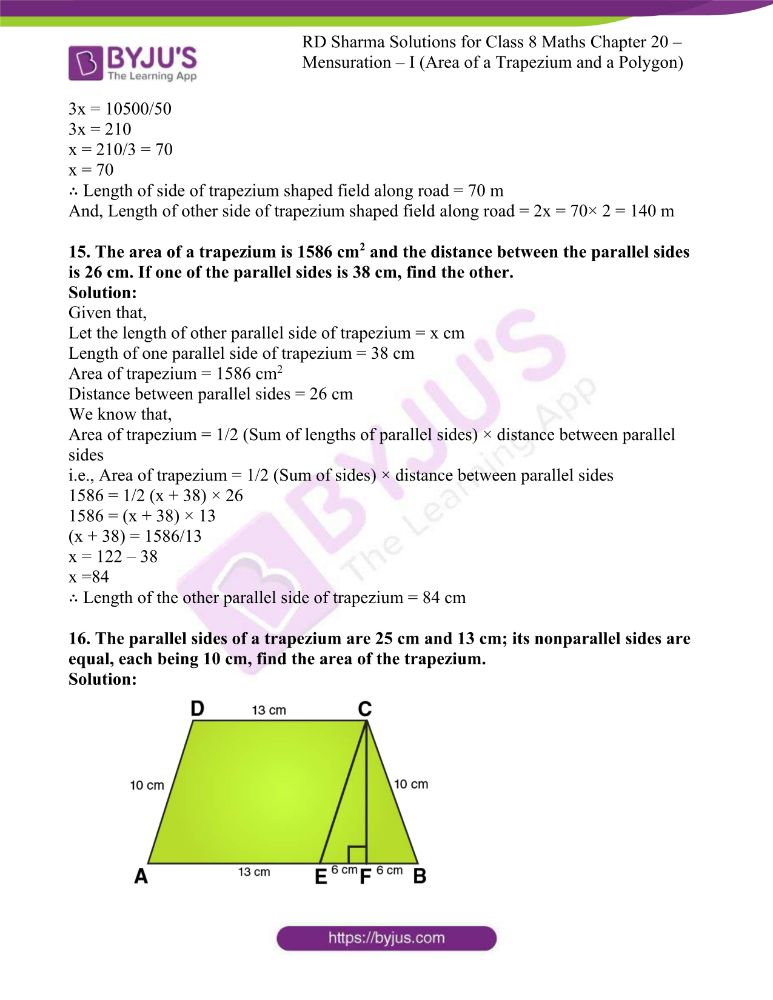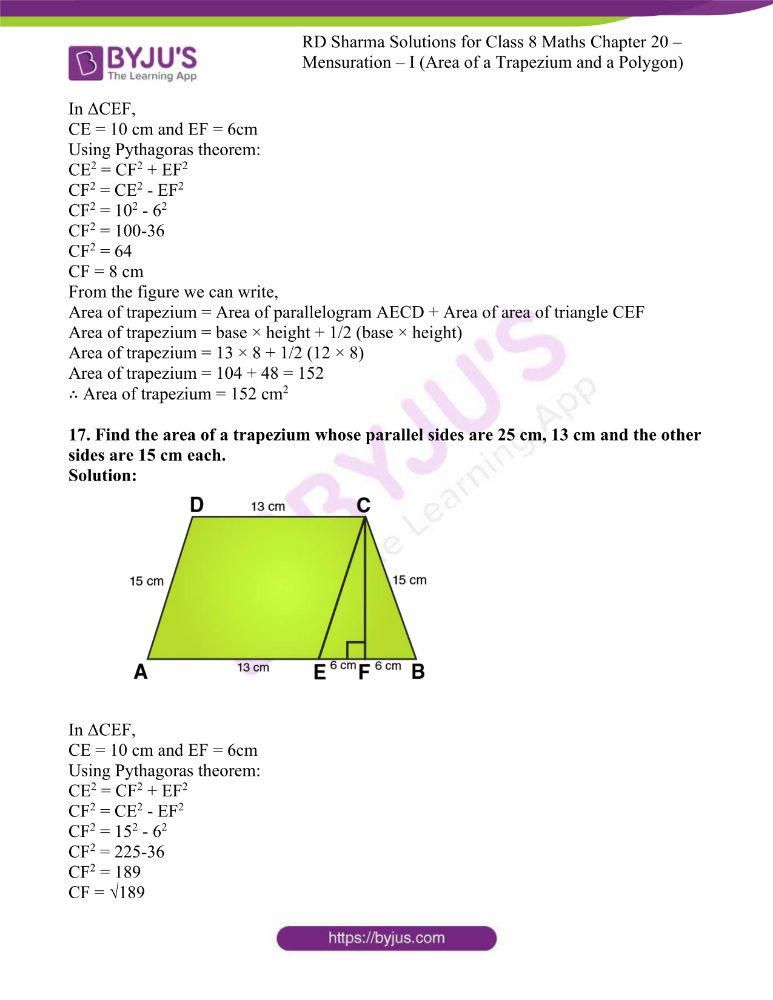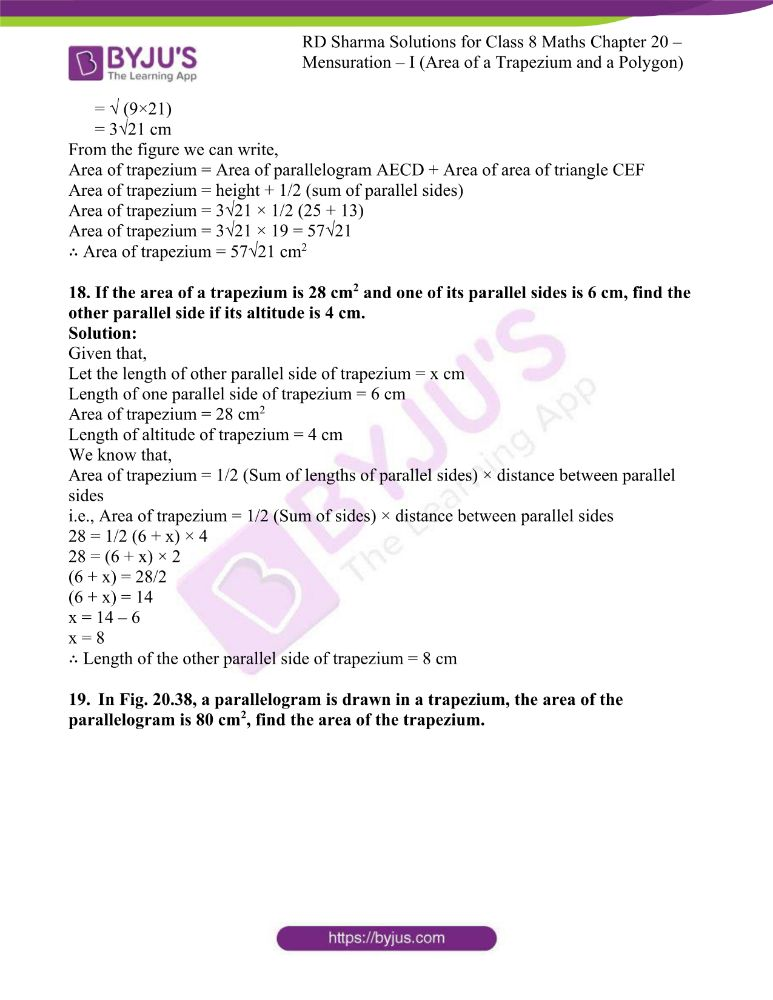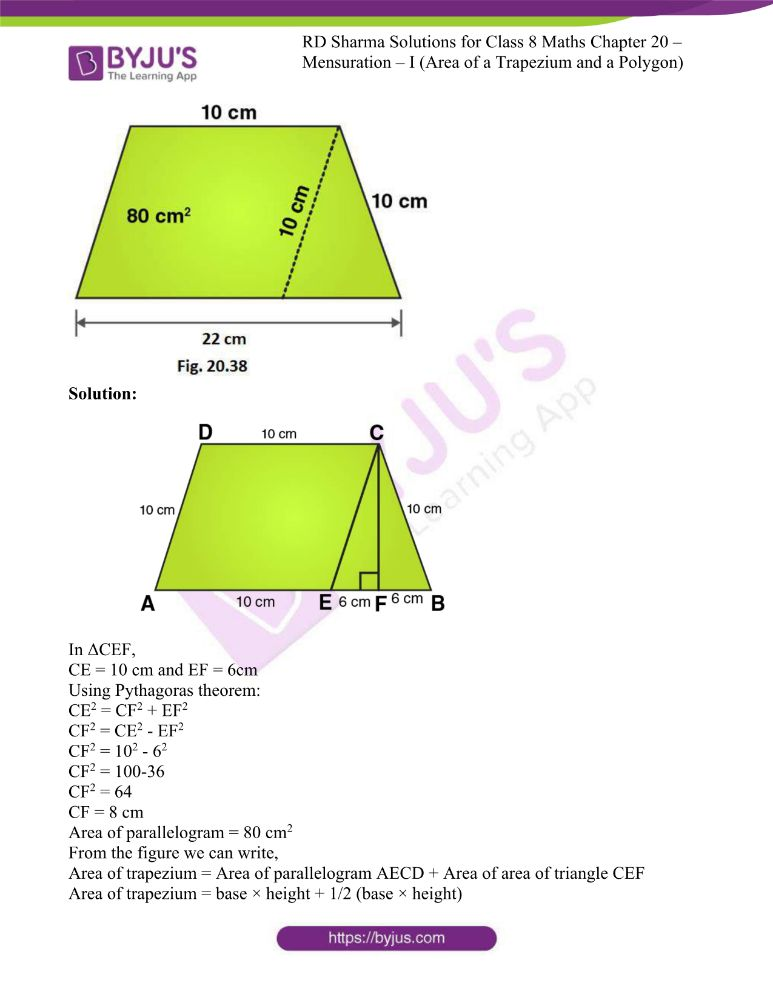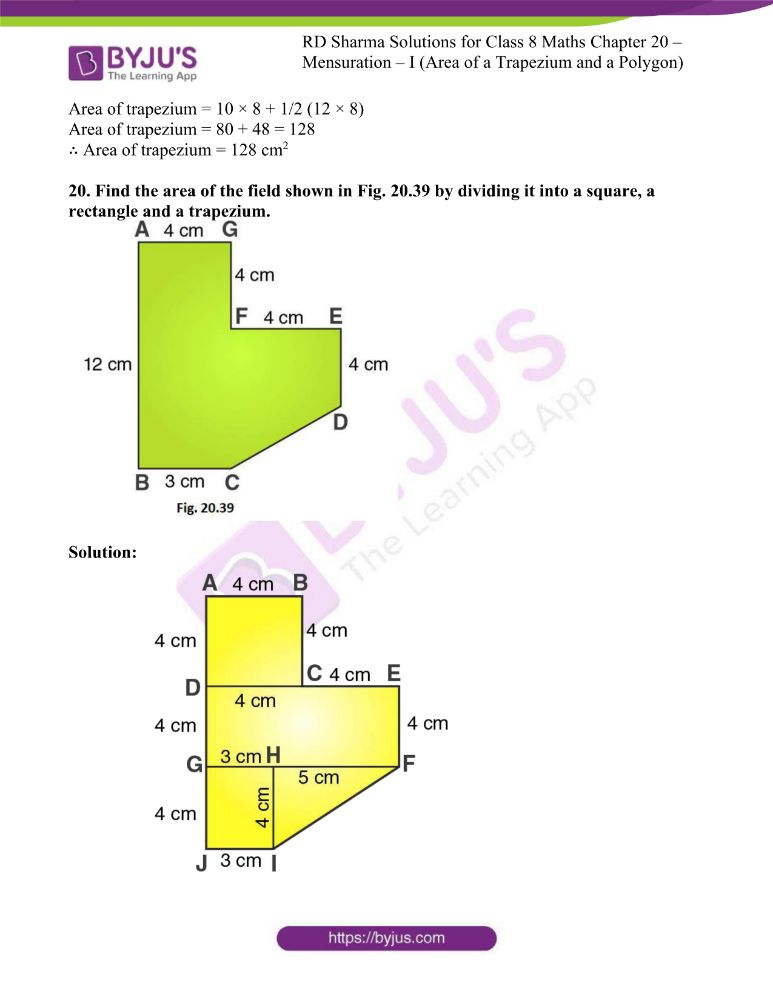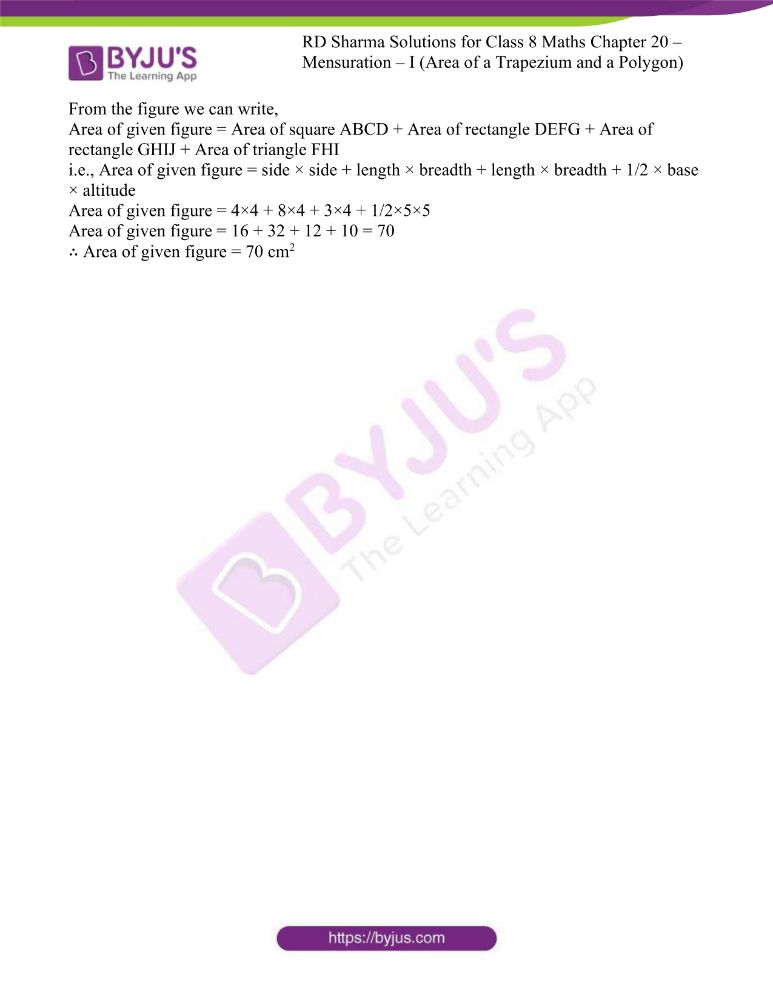### Access answers to RD Sharma Maths Solutions For Class 8 Exercise 20.2 Chapter 20 Mensuration – I (Area of a Trapezium and a Polygon)

1. Find the area, in square metres, of the trapezium whose bases and altitudes are as under:
(i) bases = 12 dm and 20 dm, altitude = 10 dm
(ii) bases = 28 cm and 3 dm, altitude = 25 cm
(iii) bases = 8 m and 60 dm, altitude = 40 dm
(iv) bases = 150 cm and 30 dm, altitude = 9 dm

Solution:

(i) Given that,

Length of bases of trapezium = 12 dm and 20 dm

Length of altitude = 10 dm

We know that, 10 dm = 1 m

∴ Length of bases in m = 1.2 m and 2 m

Similarly, length of altitude in m = 1 m

Area of trapezium = 1/2 (Sum of lengths of parallel sides) × altitude

Area of trapezium = 1/2 (1.2 + 2.0) × 1

Area of trapezium = 1/2 × 3.2 = 1.6

So, Area of trapezium = 1.6m2

(ii) Given that,

Length of bases of trapezium = 28 cm and 3 dm

Length of altitude = 25 cm

We know that, 10 dm = 1 m

∴ Length of bases in m = 0.28 m and 0.3 m

Similarly, length of altitude in m = 0.25 m

Area of trapezium = 1/2 (Sum of lengths of parallel sides) × altitude

Area of trapezium = 1/2 (0.28 + 0.3) × 0.25

Area of trapezium = 1/2 × 0.58× 0.25 = 0.0725

So, Area of trapezium = 0.0725m2

(iii) Given that,

Length of bases of trapezium = 8 m and 60 dm

Length of altitude = 40 dm

We know that, 10 dm = 1 m

∴ Length of bases in m = 8 m and 6 m

Similarly, length of altitude in m = 4 m

Area of trapezium = 1/2 (Sum of lengths of parallel sides) × altitude

Area of trapezium = 1/2 (8 + 6) × 4

Area of trapezium = 1/2 × 56 = 28

So, Area of trapezium = 28m2

(iv) Given that,

Length of bases of trapezium = 150 cm and 30 dm

Length of altitude = 9 dm

We know that, 10 dm = 1 m

∴ Length of bases in m = 1.5 m and 3 m

Similarly, length of altitude in m = 0.9 m

Area of trapezium = 1/2 (Sum of lengths of parallel sides) × altitude

Area of trapezium = 1/2 (1.5 + 3) × 0.9

Area of trapezium = 1/2 × 4.5 × 0.9 = 2.025

So, Area of trapezium = 2.025m2

2. Find the area of trapezium with base 15 cm and height 8 cm, if the side parallel to the given base is 9 cm long.

Solution:

Given that,

Length of bases of trapezium = 15 cm and 9 cm

Length of altitude = 8 cm

We know that,

Area of trapezium = 1/2 (Sum of lengths of parallel sides) × altitude

Area of trapezium = 1/2 (15 + 9) × 8

Area of trapezium = 1/2 × 192 = 96

So, Area of trapezium = 96m2

3. Find the area of a trapezium whose parallel sides are of length 16 dm and 22 dm and whose height is 12 dm.

Solution:

Given that,

Length of bases of trapezium = 16 dm and 22 dm

Length of altitude = 12 dm

We know that, 10 dm = 1 m

∴ Length of bases in m = 1.6 m and 2.2 m

Similarly, length of altitude in m = 1.2 m

Area of trapezium = 1/2 (Sum of lengths of parallel sides) × altitude

Area of trapezium = 1/2 (1.6 + 2.2) × 1.2

Area of trapezium = 1/2 × 3.8 × 1.2 = 2.28

So, Area of trapezium = 2.28m2

4. Find the height of a trapezium, the sum of the lengths of whose bases (parallel sides) is 60 cm and whose area is 600 cm2.

Solution:

Given that,

Length of bases of trapezium = 60 cm

Area = 600 cm2

We know that,

Area of trapezium = 1/2 (Sum of lengths of parallel sides) × altitude

600 = 1/2 (60) × altitude

600 = 30 × altitude

Which implies, altitude = 600/30 = 20

∴ Length of altitude is 20 cm

5. Find the altitude of a trapezium whose area is 65 cm2 and whose base are 13 cm and 26 cm.

Solution:

Given that,

Length of bases of trapezium = 13 cm and 26 cm

Area = 65 cm2

We know that,

Area of trapezium = 1/2 (Sum of lengths of parallel sides) × altitude

65 = 1/2 (13 + 26) × altitude

65 = 39/2 × altitude

Which implies, altitude = (65×2) /39 = 130/39 = 10/3

∴ Length of altitude = 10/3 cm

6. Find the sum of the lengths of the bases of a trapezium whose area is 4.2 m2 and whose height is 280 cm.

Solution:

Given that,

Height of trapezium = 280 cm = 2.8m

Area = 4.2 m2

We know that,

Area of trapezium = 1/2 (Sum of lengths of parallel sides) × altitude                                    To calculate the length of parallel sides we can rewrite the above equation as,

Sum of lengths of parallel sides = (2 × Area) / altitude

Sum of lengths of parallel sides = (2 × 4.2) / 2.8 = 8.4/2.8 = 3

∴ Sum of lengths of parallel sides = 3 m

7. Find the area of a trapezium whose parallel sides of lengths 10 cm and 15 cm are at a distance of 6 cm from each other. Calculate this area as,
(i) the sum of the areas of two triangles and one rectangle.
(ii) the difference of the area of a rectangle and the sum of the areas of two triangles.

Solution: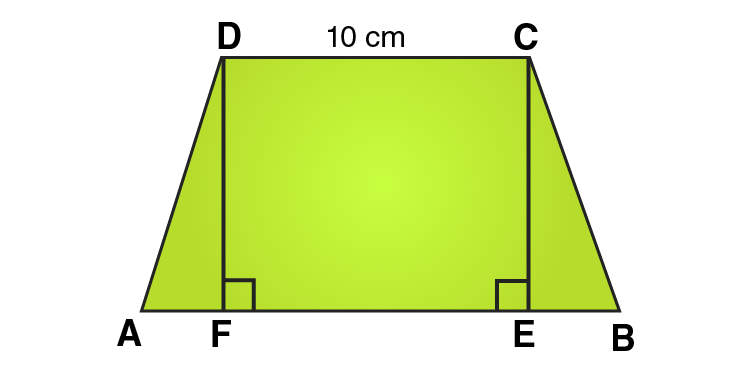We know that, Area of a trapezium ABCD

= area (∆DFA) + area (rectangle DFEC) + area (∆CEB)

= (1/2 × AF × DF) + (FE × DF) + (1/2 × EB × CE)

= (1/2 × AF × h) + (FE × h) + (1/2 × EB × h)

= 1/2 × h × (AF + 2FE + EB)

= 1/2 × h × (AF + FE + EB + FE)

= 1/2 × h × (AB + FE)

= 1/2 × h × (AB + CD) [Opposite sides of rectangle are equal]

= 1/2 × 6 × (15 + 10)

= 1/2 × 6 × 25 = 75

∴ Area of trapezium = 75 cm2

8. The area of a trapezium is 960 cm2. If the parallel sides are 34 cm and 46 cm, find the distance between them.

Solution:

We know that,

Area of trapezium = 1/2 (Sum of lengths of parallel sides) × distance between parallel sides

i.e., Area of trapezium = 1/2 (Sum of sides) × distance between parallel sides

To calculate the distance between parallel sides we can rewrite the above equation as,

Distance between parallel sides = (2 × Area) / Sum of sides

= (2 × 960) / (34 + 46)

= (2 × 960) / 80 = 1920/80 = 24

∴ Distance between parallel sides = 24 cm

9. Find the area of Fig. 20.35 as the sum of the areas of two trapezium and a rectangle.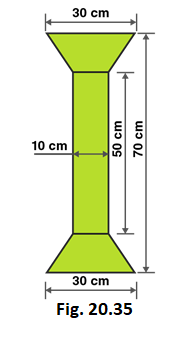Solution:

From the figure we can write,

Area of figure = Area of two trapeziums + Area of rectangle

Given that,

Length of rectangle = 50 cm

Breadth of rectangle = 10 cm

Length of parallel sides of trapezium = 30 cm and 10 cm

Distance between parallel sides of trapezium = (70–50)/2 = 20/2 = 10

So, Distance between parallel sides of trapezium = 10 cm

Area of figure = 2 × 1/2 (Sum of lengths of parallel sides) × altitude + Length × Breadth

Area of figure = 2 × 1/2 (30+10) × 10 + 50 × 10

Area of figure = 40 × 10 + 50 × 10

Area of figure = 400 + 500 = 900

∴ Area of figure = 900 cm2

10. Top surface of a table is trapezium in shape. Find its area if its parallel sides are 1 m and 1.2 m and perpendicular distance between them is 0.8 m.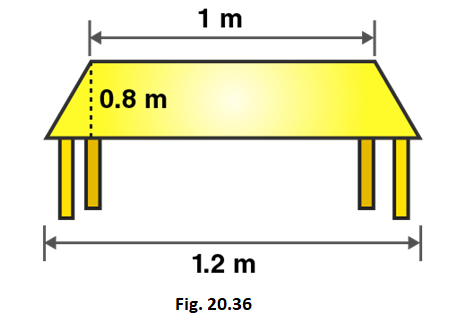Solution:

Given that,

Length of parallel sides of trapezium = 1.2m and 1m

Distance between parallel sides of trapezium = 0.8m

We know that,

Area of trapezium = 1/2 (Sum of lengths of parallel sides) × distance between parallel sides

i.e., Area of trapezium = 1/2 (Sum of sides) × distance between parallel sides

Area of trapezium = 1/2 (1.2 + 1) × 0.8

Area of trapezium = 1/2 × 2.2 × 0.8 = 0.88

So, Area of trapezium = 0.88m2

11. The cross-section of a canal is a trapezium in shape. If the canal is 10 m wide at the top 6 m wide at the bottom and the area of cross-section is 72 m2 determine its depth.

Solution:

Given that,

Length of parallel sides of trapezium = 10m and 6m

Area = 72 m2

Let the distance between parallel sides of trapezium = x meter

We know that,

Area of trapezium = 1/2 (Sum of lengths of parallel sides) × distance between parallel sides

i.e., Area of trapezium = 1/2 (Sum of sides) × distance between parallel sides

72 = 1/2 (10 + 6) × x

72 = 8 × x

x = 72/8 = 9

∴ The depth is 9m.

12. The area of a trapezium is 91 cm2 and its height is 7 cm. If one of the parallel sides is longer than the other by 8 cm, find the two parallel sides.

Solution:

Given that,

Let the length of one parallel side of trapezium = x meter

Length of other parallel side of trapezium = (x+8) meter

Area of trapezium = 91 cm2

Height = 7 cm

We know that,

Area of trapezium = 1/2 (Sum of lengths of parallel sides) × altitude

91 = 1/2 (x+x+8) × 7

91 = 1/2(2x+8) × 7

91 = (x+4) × 7

(x+4) = 91/7

x+4 = 13

x = 13 – 4

x = 9

∴ Length of one parallel side of trapezium = 9 cm

And, Length of other parallel side of trapezium = x+8 = 9+8 = 17 cm

13. The area of a trapezium is 384 cm2. Its parallel sides are in the ratio 3:5 and the perpendicular distance between them is 12 cm. Find the length of each one of the parallel sides.

Solution:

Given that,

Let the length of one parallel side of trapezium = 3x meter

Length of other parallel side of trapezium = 5x meter

Area of trapezium = 384 cm2

Distance between parallel sides = 12 cm

We know that,

Area of trapezium = 1/2 (Sum of lengths of parallel sides) × distance between parallel sides

i.e., Area of trapezium = 1/2 (Sum of sides) × distance between parallel sides

384 = 1/2 (3x + 5x) × 12

384 = 1/2 (8x) × 12

4x = 384/12

4x = 32

x = 8

∴ Length of one parallel side of trapezium = 3x = 3× 8 = 24 cm

And, Length of other parallel side of trapezium = 5x = 5× 8 = 40 cm

14. Mohan wants to buy a trapezium shaped field. Its side along the river is parallel and twice the side along the road. If the area of this field is 10500 m2 and the perpendicular distance between the two parallel sides is 100 m, find the length of the side along the river.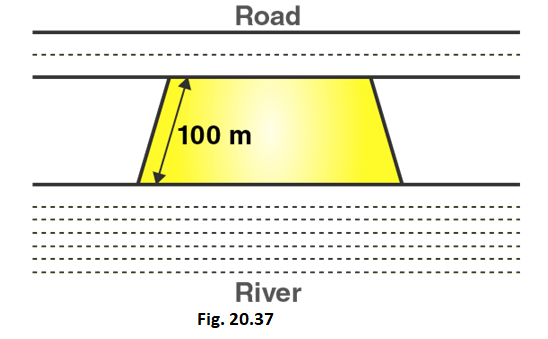Solution:

Given that,

Let the length of side of trapezium shaped field along road = x meter

Length of other side of trapezium shaped field along road = 2x meter

Area of trapezium = 10500 cm2

Distance between parallel sides = 100 m

We know that,

Area of trapezium = 1/2 (Sum of lengths of parallel sides) × distance between parallel sides

i.e., Area of trapezium = 1/2 (Sum of sides) × distance between parallel sides

10500 = 1/2 (x + 2x) × 100

10500 = 1/2 (3x) × 100

3x = 10500/50

3x = 210

x = 210/3 = 70

x = 70

∴ Length of side of trapezium shaped field along road = 70 m

And, Length of other side of trapezium shaped field along road = 2x = 70× 2 = 140 m

15. The area of a trapezium is 1586 cm2 and the distance between the parallel sides is 26 cm. If one of the parallel sides is 38 cm, find the other.

Solution:

Given that,

Let the length of other parallel side of trapezium = x cm

Length of one parallel side of trapezium = 38 cm

Area of trapezium = 1586 cm2

Distance between parallel sides = 26 cm

We know that,

Area of trapezium = 1/2 (Sum of lengths of parallel sides) × distance between parallel sides

i.e., Area of trapezium = 1/2 (Sum of sides) × distance between parallel sides

1586 = 1/2 (x + 38) × 26

1586 = (x + 38) × 13

(x + 38) = 1586/13

x = 122 – 38

x =84

∴ Length of the other parallel side of trapezium = 84 cm

16. The parallel sides of a trapezium are 25 cm and 13 cm; its nonparallel sides are equal, each being 10 cm, find the area of the trapezium.

Solution: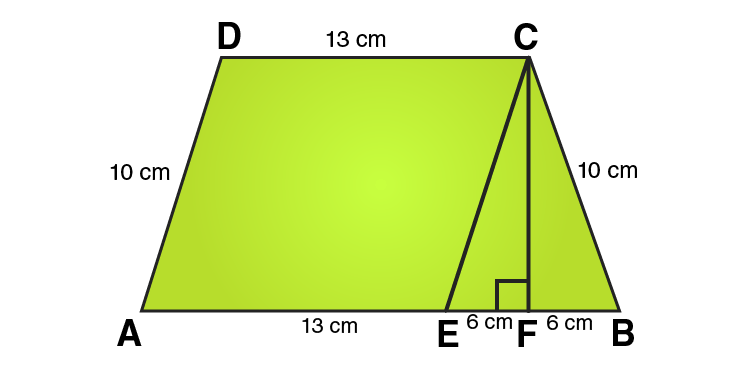In ΔCEF,

CE = 10 cm and EF = 6cm

Using Pythagoras theorem:

CE2 = CF2 + EF2

CF2 = CE2 – EF2

CF2 = 102 – 62

CF2 = 100-36

CF2 = 64

CF = 8 cm

From the figure we can write,

Area of trapezium = Area of parallelogram AECD + Area of area of triangle CEF

Area of trapezium = base × height + 1/2 (base × height)

Area of trapezium = 13 × 8 + 1/2 (12 × 8)

Area of trapezium = 104 + 48 = 152

∴ Area of trapezium = 152 cm2

17. Find the area of a trapezium whose parallel sides are 25 cm, 13 cm and the other sides are 15 cm each.

Solution: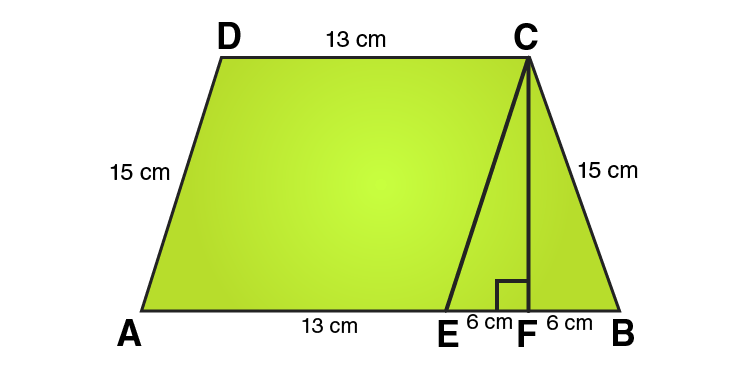In ΔCEF,

CE = 10 cm and EF = 6cm

Using Pythagoras theorem:

CE2 = CF2 + EF2

CF2 = CE2 – EF2

CF2 = 152 – 62

CF2 = 225-36

CF2 = 189

CF = √189

= √ (9×21)

= 3√21 cm

From the figure we can write,

Area of trapezium = Area of parallelogram AECD + Area of area of triangle CEF

Area of trapezium = height + 1/2 (sum of parallel sides)

Area of trapezium = 3√21 × 1/2 (25 + 13)

Area of trapezium = 3√21 × 19 = 57√21

∴ Area of trapezium = 57√21 cm2

18. If the area of a trapezium is 28 cm2 and one of its parallel sides is 6 cm, find the other parallel side if its altitude is 4 cm.

Solution:

Given that,

Let the length of other parallel side of trapezium = x cm

Length of one parallel side of trapezium = 6 cm

Area of trapezium = 28 cm2

Length of altitude of trapezium = 4 cm

We know that,

Area of trapezium = 1/2 (Sum of lengths of parallel sides) × distance between parallel sides

i.e., Area of trapezium = 1/2 (Sum of sides) × distance between parallel sides

28 = 1/2 (6 + x) × 4

28 = (6 + x) × 2

(6 + x) = 28/2

(6 + x) = 14

x = 14 – 6

x = 8

∴ Length of the other parallel side of trapezium = 8 cm

19. In Fig. 20.38, a parallelogram is drawn in a trapezium, the area of the parallelogram is 80 cm2, find the area of the trapezium.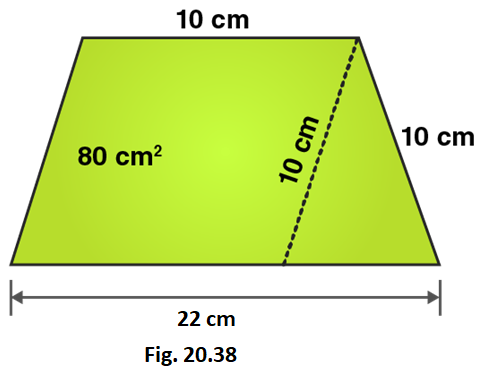Solution: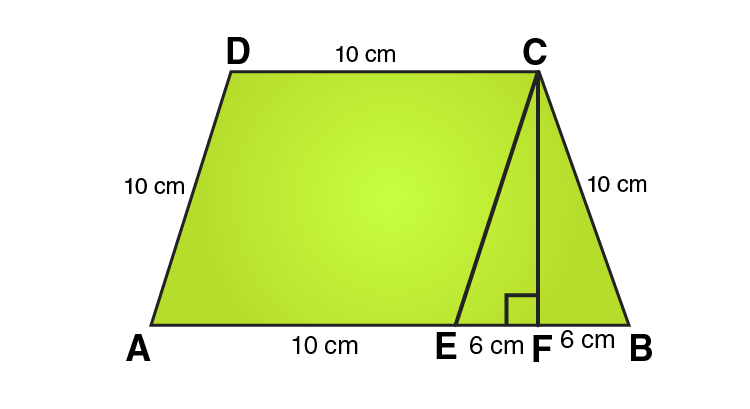In ΔCEF,

CE = 10 cm and EF = 6cm

Using Pythagoras theorem:

CE2 = CF2 + EF2

CF2 = CE2 – EF2

CF2 = 102 – 62

CF2 = 100-36

CF2 = 64

CF = 8 cm

Area of parallelogram = 80 cm2

From the figure we can write,

Area of trapezium = Area of parallelogram AECD + Area of area of triangle CEF

Area of trapezium = base × height + 1/2 (base × height)

Area of trapezium = 10 × 8 + 1/2 (12 × 8)

Area of trapezium = 80 + 48 = 128

∴ Area of trapezium = 128 cm2

20. Find the area of the field shown in Fig. 20.39 by dividing it into a square, a rectangle and a trapezium.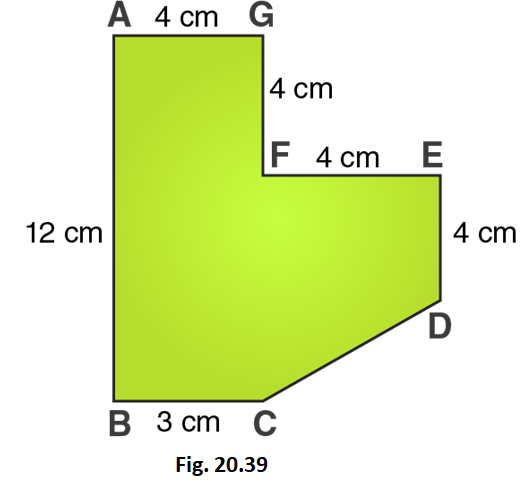Solution: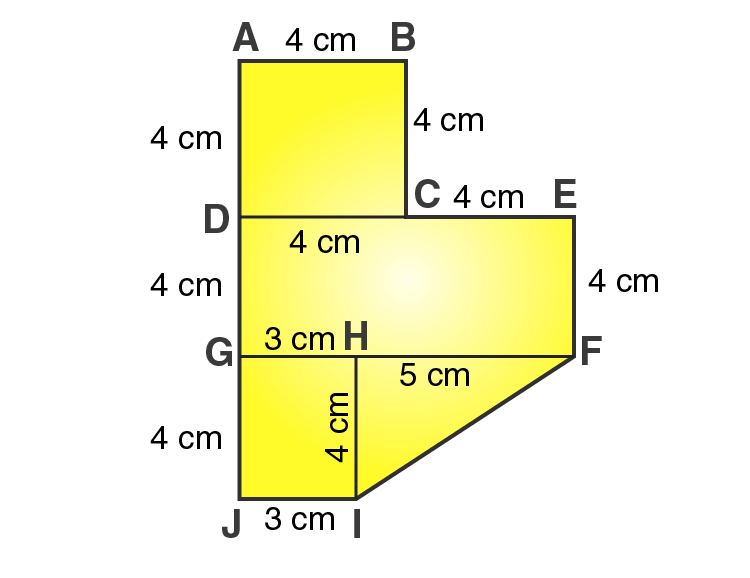From the figure we can write,

Area of given figure = Area of square ABCD + Area of rectangle DEFG + Area of rectangle GHIJ + Area of triangle FHI

i.e., Area of given figure = side × side + length × breadth + length × breadth + 1/2 × base × altitude

Area of given figure = 4×4 + 8×4 + 3×4 + 1/2×5×5

Area of given figure = 16 + 32 + 12 + 10 = 70

∴ Area of given figure = 70 cm2